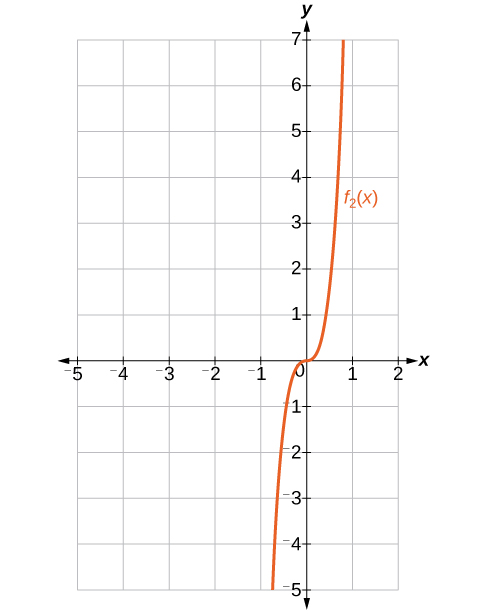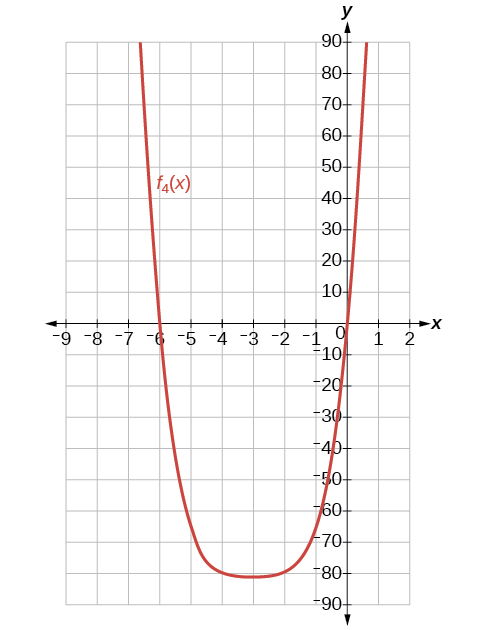# 13.6 Binomial theorem  (Page 3/6)

 Page 3 / 6

## Key equations

 Binomial Theorem ${\left(x+y\right)}^{n}=\sum _{k-0}^{n}\left(\begin{array}{c}n\\ k\end{array}\right){x}^{n-k}{y}^{k}$ $\left(r+1\right)th\text{\hspace{0.17em}}$ term of a binomial expansion $\left(\begin{array}{c}n\\ r\end{array}\right){x}^{n-r}{y}^{r}$

## Key concepts

• $\left(\begin{array}{c}n\\ r\end{array}\right)\text{\hspace{0.17em}}$ is called a binomial coefficient and is equal to $C\left(n,r\right).\text{\hspace{0.17em}}$ See [link] .
• The Binomial Theorem allows us to expand binomials without multiplying. See [link] .
• We can find a given term of a binomial expansion without fully expanding the binomial. See [link] .

## Verbal

What is a binomial coefficient, and how it is calculated?

A binomial coefficient is an alternative way of denoting the combination $\text{\hspace{0.17em}}C\left(n,r\right).\text{\hspace{0.17em}}$ It is defined as $\text{\hspace{0.17em}}\left(\begin{array}{c}n\\ r\end{array}\right)=\text{\hspace{0.17em}}C\left(n,r\right)\text{\hspace{0.17em}}=\frac{n!}{r!\left(n-r\right)!}.$

What role do binomial coefficients play in a binomial expansion? Are they restricted to any type of number?

What is the Binomial Theorem and what is its use?

The Binomial Theorem is defined as $\text{\hspace{0.17em}}{\left(x+y\right)}^{n}=\sum _{k=0}^{n}\left(\begin{array}{c}n\\ k\end{array}\right){x}^{n-k}{y}^{k}\text{\hspace{0.17em}}$ and can be used to expand any binomial.

When is it an advantage to use the Binomial Theorem? Explain.

## Algebraic

For the following exercises, evaluate the binomial coefficient.

$\left(\begin{array}{c}6\\ 2\end{array}\right)$

15

$\left(\begin{array}{c}5\\ 3\end{array}\right)$

$\left(\begin{array}{c}7\\ 4\end{array}\right)$

35

$\left(\begin{array}{c}9\\ 7\end{array}\right)$

$\left(\begin{array}{c}10\\ 9\end{array}\right)$

10

$\left(\begin{array}{c}25\\ 11\end{array}\right)$

$\left(\begin{array}{c}17\\ 6\end{array}\right)$

12,376

$\left(\begin{array}{c}200\\ 199\end{array}\right)$

For the following exercises, use the Binomial Theorem to expand each binomial.

${\left(4a-b\right)}^{3}$

$64{a}^{3}-48{a}^{2}b+12a{b}^{2}-{b}^{3}$

${\left(5a+2\right)}^{3}$

${\left(3a+2b\right)}^{3}$

$27{a}^{3}+54{a}^{2}b+36a{b}^{2}+8{b}^{3}$

${\left(2x+3y\right)}^{4}$

${\left(4x+2y\right)}^{5}$

$1024{x}^{5}+2560{x}^{4}y+2560{x}^{3}{y}^{2}+1280{x}^{2}{y}^{3}+320x{y}^{4}+32{y}^{5}$

${\left(3x-2y\right)}^{4}$

${\left(4x-3y\right)}^{5}$

$1024{x}^{5}-3840{x}^{4}y+5760{x}^{3}{y}^{2}-4320{x}^{2}{y}^{3}+1620x{y}^{4}-243{y}^{5}$

${\left(\frac{1}{x}+3y\right)}^{5}$

${\left({x}^{-1}+2{y}^{-1}\right)}^{4}$

$\frac{1}{{x}^{4}}+\frac{8}{{x}^{3}y}+\frac{24}{{x}^{2}{y}^{2}}+\frac{32}{x{y}^{3}}+\frac{16}{{y}^{4}}$

${\left(\sqrt{x}-\sqrt{y}\right)}^{5}$

For the following exercises, use the Binomial Theorem to write the first three terms of each binomial.

${\left(a+b\right)}^{17}$

${a}^{17}+17{a}^{16}b+136{a}^{15}{b}^{2}$

${\left(x-1\right)}^{18}$

${\left(a-2b\right)}^{15}$

${a}^{15}-30{a}^{14}b+420{a}^{13}{b}^{2}$

${\left(x-2y\right)}^{8}$

${\left(3a+b\right)}^{20}$

$3,486,784,401{a}^{20}+23,245,229,340{a}^{19}b+73,609,892,910{a}^{18}{b}^{2}$

${\left(2a+4b\right)}^{7}$

${\left({x}^{3}-\sqrt{y}\right)}^{8}$

${x}^{24}-8{x}^{21}\sqrt{y}+28{x}^{18}y$

For the following exercises, find the indicated term of each binomial without fully expanding the binomial.

The fourth term of $\text{\hspace{0.17em}}{\left(2x-3y\right)}^{4}$

The fourth term of $\text{\hspace{0.17em}}{\left(3x-2y\right)}^{5}$

$-720{x}^{2}{y}^{3}$

The third term of $\text{\hspace{0.17em}}{\left(6x-3y\right)}^{7}$

The eighth term of $\text{\hspace{0.17em}}{\left(7+5y\right)}^{14}$

$220,812,466,875,000{y}^{7}$

The seventh term of $\text{\hspace{0.17em}}{\left(a+b\right)}^{11}$

The fifth term of $\text{\hspace{0.17em}}{\left(x-y\right)}^{7}$

$35{x}^{3}{y}^{4}$

The tenth term of $\text{\hspace{0.17em}}{\left(x-1\right)}^{12}$

The ninth term of $\text{\hspace{0.17em}}{\left(a-3{b}^{2}\right)}^{11}$

$1,082,565{a}^{3}{b}^{16}$

The fourth term of $\text{\hspace{0.17em}}{\left({x}^{3}-\frac{1}{2}\right)}^{10}$

The eighth term of $\text{\hspace{0.17em}}{\left(\frac{y}{2}+\frac{2}{x}\right)}^{9}$

$\frac{1152{y}^{2}}{{x}^{7}}$

## Graphical

For the following exercises, use the Binomial Theorem to expand the binomial $f\left(x\right)={\left(x+3\right)}^{4}.$ Then find and graph each indicated sum on one set of axes.

Find and graph $\text{\hspace{0.17em}}{f}_{1}\left(x\right),\text{\hspace{0.17em}}$ such that $\text{\hspace{0.17em}}{f}_{1}\left(x\right)\text{\hspace{0.17em}}$ is the first term of the expansion.

Find and graph $\text{\hspace{0.17em}}{f}_{2}\left(x\right),\text{\hspace{0.17em}}$ such that $\text{\hspace{0.17em}}{f}_{2}\left(x\right)\text{\hspace{0.17em}}$ is the sum of the first two terms of the expansion.

${f}_{2}\left(x\right)={x}^{4}+12{x}^{3}$Find and graph $\text{\hspace{0.17em}}{f}_{3}\left(x\right),\text{\hspace{0.17em}}$ such that $\text{\hspace{0.17em}}{f}_{3}\left(x\right)\text{\hspace{0.17em}}$ is the sum of the first three terms of the expansion.

Find and graph $\text{\hspace{0.17em}}{f}_{4}\left(x\right),\text{\hspace{0.17em}}$ such that $\text{\hspace{0.17em}}{f}_{4}\left(x\right)\text{\hspace{0.17em}}$ is the sum of the first four terms of the expansion.

${f}_{4}\left(x\right)={x}^{4}+12{x}^{3}+54{x}^{2}+108x$Find and graph $\text{\hspace{0.17em}}{f}_{5}\left(x\right),\text{\hspace{0.17em}}$ such that $\text{\hspace{0.17em}}{f}_{5}\left(x\right)\text{\hspace{0.17em}}$ is the sum of the first five terms of the expansion.

## Extensions

In the expansion of $\text{\hspace{0.17em}}{\left(5x+3y\right)}^{n},\text{\hspace{0.17em}}$ each term has the form successively takes on the value $\text{\hspace{0.17em}}0,1,2,\text{\hspace{0.17em}}...,\text{\hspace{0.17em}}n.$ If $\text{\hspace{0.17em}}\left(\begin{array}{c}n\\ k\end{array}\right)=\left(\begin{array}{c}7\\ 2\end{array}\right),\text{\hspace{0.17em}}$ what is the corresponding term?

$590,625{x}^{5}{y}^{2}$

In the expansion of $\text{\hspace{0.17em}}{\left(a+b\right)}^{n},\text{\hspace{0.17em}}$ the coefficient of $\text{\hspace{0.17em}}{a}^{n-k}{b}^{k}\text{\hspace{0.17em}}$ is the same as the coefficient of which other term?

Consider the expansion of $\text{\hspace{0.17em}}{\left(x+b\right)}^{40}.\text{\hspace{0.17em}}$ What is the exponent of $b$ in the $k\text{th}$ term?

$k-1$

Find $\text{\hspace{0.17em}}\left(\begin{array}{c}n\\ k-1\end{array}\right)+\left(\begin{array}{c}n\\ k\end{array}\right)\text{\hspace{0.17em}}$ and write the answer as a binomial coefficient in the form $\text{\hspace{0.17em}}\left(\begin{array}{c}n\\ k\end{array}\right).\text{\hspace{0.17em}}$ Prove it. Hint: Use the fact that, for any integer $\text{\hspace{0.17em}}p,\text{\hspace{0.17em}}$ such that $\text{\hspace{0.17em}}p\ge 1,\text{\hspace{0.17em}}p!=p\left(p-1\right)!\text{.}$

$\left(\begin{array}{c}n\\ k-1\end{array}\right)+\left(\begin{array}{l}n\\ k\end{array}\right)=\left(\begin{array}{c}n+1\\ k\end{array}\right);\text{\hspace{0.17em}}$ Proof:

$\begin{array}{}\\ \\ \\ \text{\hspace{0.17em}}\text{\hspace{0.17em}}\text{\hspace{0.17em}}\text{\hspace{0.17em}}\text{\hspace{0.17em}}\left(\begin{array}{c}n\\ k-1\end{array}\right)+\left(\begin{array}{l}n\\ k\end{array}\right)\\ =\frac{n!}{k!\left(n-k\right)!}+\frac{n!}{\left(k-1\right)!\left(n-\left(k-1\right)\right)!}\\ =\frac{n!}{k!\left(n-k\right)!}+\frac{n!}{\left(k-1\right)!\left(n-k+1\right)!}\\ =\frac{\left(n-k+1\right)n!}{\left(n-k+1\right)k!\left(n-k\right)!}+\frac{kn!}{k\left(k-1\right)!\left(n-k+1\right)!}\\ =\frac{\left(n-k+1\right)n!+kn!}{k!\left(n-k+1\right)!}\\ =\frac{\left(n+1\right)n!}{k!\left(\left(n+1\right)-k\right)!}\\ =\frac{\left(n+1\right)!}{k!\left(\left(n+1\right)-k\right)!}\\ =\left(\begin{array}{c}n+1\\ k\end{array}\right)\end{array}$

Which expression cannot be expanded using the Binomial Theorem? Explain.

• $\left({x}^{2}-2x+1\right)$
• ${\left(\sqrt{a}+4\sqrt{a}-5\right)}^{8}$
• ${\left({x}^{3}+2{y}^{2}-z\right)}^{5}$
• ${\left(3{x}^{2}-\sqrt{2{y}^{3}}\right)}^{12}$

The expression $\text{\hspace{0.17em}}{\left({x}^{3}+2{y}^{2}-z\right)}^{5}\text{\hspace{0.17em}}$ cannot be expanded using the Binomial Theorem because it cannot be rewritten as a binomial.

By the definition, is such that 0!=1.why?
(1+cosA+IsinA)(1+cosB+isinB)/(cos@+isin@)(cos$+isin$)
hatdog
Mark
how we can draw three triangles of distinctly different shapes. All the angles will be cutt off each triangle and placed side by side with vertices touching
bsc F. y algebra and trigonometry pepper 2
given that x= 3/5 find sin 3x
4
DB
remove any signs and collect terms of -2(8a-3b-c)
-16a+6b+2c
Will
Joeval
(x2-2x+8)-4(x2-3x+5)
sorry
Miranda
x²-2x+9-4x²+12x-20 -3x²+10x+11
Miranda
x²-2x+9-4x²+12x-20 -3x²+10x+11
Miranda
(X2-2X+8)-4(X2-3X+5)=0 ?
master
The anwser is imaginary number if you want to know The anwser of the expression you must arrange The expression and use quadratic formula To find the answer
master
The anwser is imaginary number if you want to know The anwser of the expression you must arrange The expression and use quadratic formula To find the answer
master
Y
master
master
Soo sorry (5±Root11* i)/3
master
Mukhtar
2x²-6x+1=0
Ife
explain and give four example of hyperbolic function
What is the correct rational algebraic expression of the given "a fraction whose denominator is 10 more than the numerator y?
y/y+10
Mr
Find nth derivative of eax sin (bx + c).
Find area common to the parabola y2 = 4ax and x2 = 4ay.
Anurag
y2=4ax= y=4ax/2. y=2ax
akash
A rectangular garden is 25ft wide. if its area is 1125ft, what is the length of the garden
to find the length I divide the area by the wide wich means 1125ft/25ft=45
Miranda
thanks
Jhovie
What do you call a relation where each element in the domain is related to only one value in the range by some rules?
A banana.
Yaona
a function
Daniel
a function
emmanuel
given 4cot thither +3=0and 0°<thither <180° use a sketch to determine the value of the following a)cos thither
what are you up to?
nothing up todat yet
Miranda
hi
jai
hello
jai
Miranda Drice
jai
aap konsi country se ho
jai
which language is that
Miranda
I am living in india
jai
good
Miranda
what is the formula for calculating algebraic
I think the formula for calculating algebraic is the statement of the equality of two expression stimulate by a set of addition, multiplication, soustraction, division, raising to a power and extraction of Root. U believe by having those in the equation you will be in measure to calculate it
Miranda

#### Get Jobilize Job Search Mobile App in your pocket Now!ByByBy OpenStaxBy OpenStaxBy OpenStaxBy Yacoub JayoghliBy Brooke DelaneyBy Samuel MaddenBy Brooke DelaneyBy Brooke DelaneyBy Darlene PaliswatBy OpenStax